Next: MIDAS utilities for time Up: Fourier analysis: The sine Previous: The power spectrum and

## Sampling patterns

The effect of a certain sampling pattern in the frequency analysis is particularly transparent for the power spectrum. Let s be the sampling function taking on the value 1 at the (unevenly spaced) times of the observations observation and 0 elsewhere. The power spectrum of the sampling function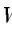=(12.11)

is an ordinary, non-random function called the spectral window function. The discrete observations are the product of s and the model function f: x = s f so that their transform is a convolution of transforms: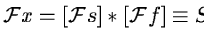, where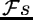and. For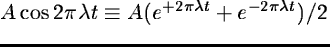and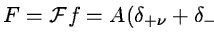we obtain the result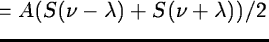. Because of the linearity of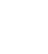our result extends to any combination of frequencies. Taking the square modulus of the result equation, we obtain both squared and mixed terms. The mixed terms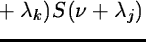correspond to an interference of frequenciesand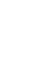differing by either sign or absolute value. Therefore, if interference between frequencies is small, the power spectrum reduces to the sum of the window functions shifted in frequency: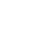(12.12)

In the opposite case of strong interference, ghost patterns may arise in the power spectrum due to interference of window function patterns belonging to positive as well as negative frequencies. The ghost patterns produced at frequencies nearby or far from the true frequency are called aliases and power leaks, respectively.Next: MIDAS utilities for time Up: Fourier analysis: The sine Previous: The power spectrum and
Petra Nass
1999-06-15